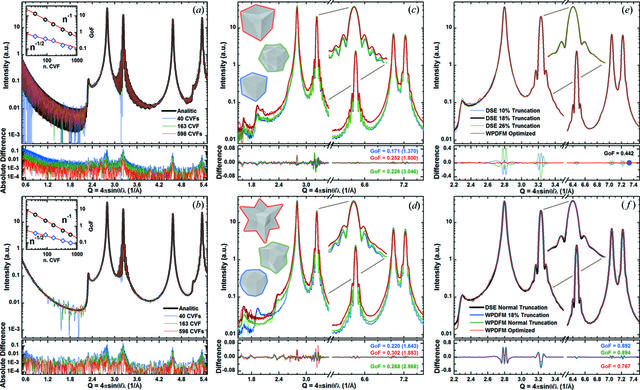disable zoom     view article Figure 6 Modeling the scattering profiles from Pd (f.c.c.) nanocrystals with arbitrary shape and shape dispersion. (a), (b) Intensity profiles from powders of cubic crystals with (a) constant and (b) lognormal size distribution (mean = 22 nm and s.d. = 1 nm), which were modeled using an increasing number of tabulated CVFs. The profiles are compared against the solution using the analytical CVF expressions. The insets show the GoF of the agreement between the patterns modeled using the analytical and the tabulated CVFs calculated in the Q range from 2.0 to 10.0 Å−1 (blue, open diamond) or including the small-angle region for Q ≤ 2.0 Å−1 (black, open circle). The exponential fits are also shown (red, line). (c), (d) Intensity profiles for powders of polyhedral crystals with the same volume (i.e. about the same number of atoms) bounded only by (c) 310 and (d) 411 facets, which were modeled using 163 tabulated CVFs. (e), (f) Intensity profiles from powders of truncated cubic crystals with lognormal size distribution (mean ≃ 14 nm and s.d. = 2.2 nm), and (e) constant and (f) normal truncation distribution (mean = 18% and s.d. = 4%). The profiles are compared with the Debye solution and the GoF of the agreement was calculated in the Q range from 2.0 to 10.0 Å−1 [the values calculated including the small-angle region (Q ≤ 2.0 Å−1) are shown in parentheses].

IUCrJ
Volume 8| Part 2| March 2021| Pages 257-269
ISSN: 2052-2525